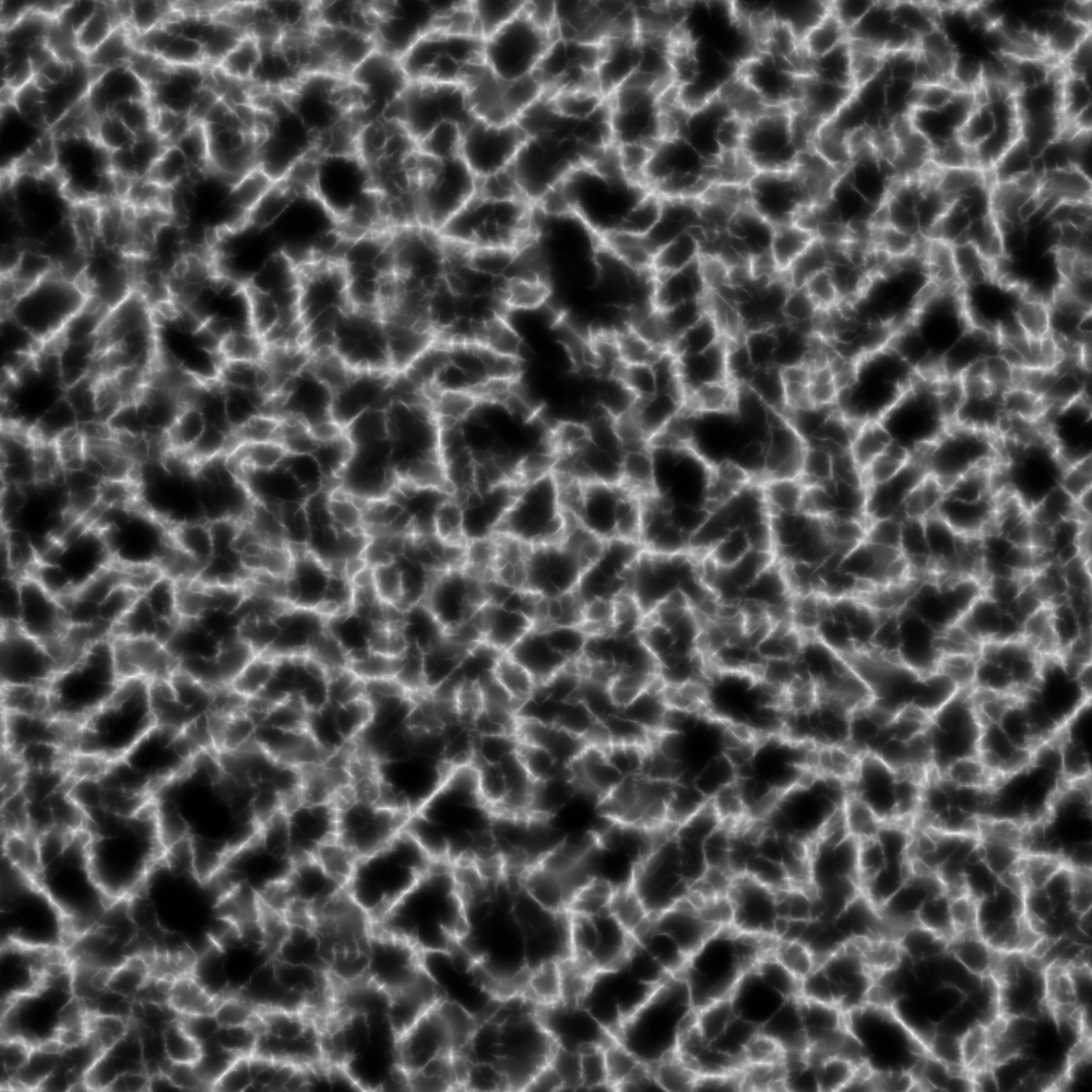This fractal is slightly more complex than the regular fbm() fractal. It uses the prior octave to modify the values of the current octave before adding it to the cumulating values. The result of this is that the final values will show steep hills and larger smooth areas, resembling mountain ranges. This function is intended to be used in conjunction with fracture()

ridged(base, new, strength, octave, offset = 1, gain = 2, ...)

spectral_gain(h = 1, lacunarity = 2)

## Arguments

base The prior values to modify The new values to modify base with A value to modify new with before applying it to base The current octave The new values are first modified by (offset - abs(new))^2 A value to multiply the old octave by before using it to modify the new octave ignored Each successive gain is raised to the power of -h A multiplier to apply to the previous value before raising it to the power of -h

## Details

The ridged fractal was designed with a slightly more complex gain sequence in mind, and while any sequence or generator would work fracture() should be called with gain = spectral_gain() to mimick the original intention of the fractal.

Other Fractal functions: billow(), clamped(), fbm()
grid <- long_grid(seq(1, 10, length.out = 1000), seq(1, 10, length.out = 1000))
grid$simplex <- fracture(gen_simplex, ridged, octaves = 8, gain = spectral_gain(), x = grid$x, y = grid\$y)